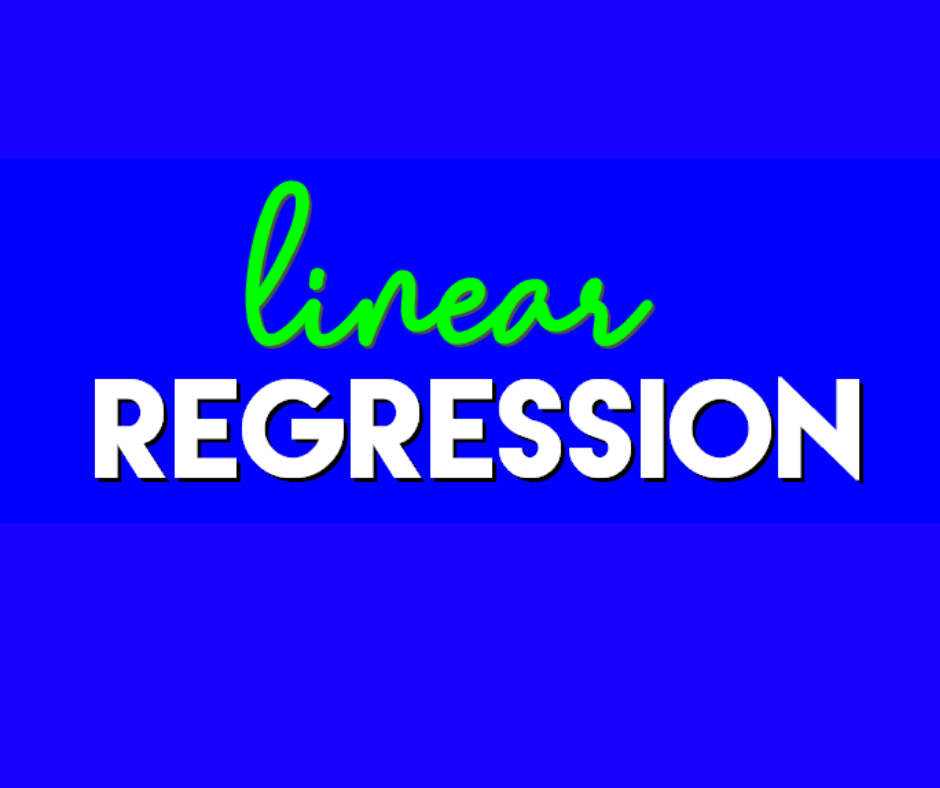top of page
Search
•Nucot

# Linear RegressionWhat is Linear Regression?

Linear Regression is a statistical way of measuring the relationship between variables to understand the trends in the Data. Linear Regression comes under the category of supervised learning algorithms in machine learning.

How does Linear Regression work?

Linear regression is the process of identifying a line that best matches the data points on the plot so that we can use it to forecast output values for inputs that aren't present in the data set we have, with the assumption that those outputs will fall on the line. It works on the principle of ordinary least square (OLS)(OLS) / Mean square error (MSE)(MSE). In statistics Ols is a method to estimate the Unkown parameter of the linear regression function, its goal is to minimize the sum of the square difference between observed dependent variable in the given data set and those predicted by linear regression faction.

Types of Linear Regression?

Regression techniques are one of the most popular statistical techniques used for predictive modelling and data mining tasks. On average, analytics professionals know only 2-3 types of regression that are commonly used in the real world. They are linear and logistic regression. But the fact is there are many regression algorithms designed for various types of analysis. Each type has its own significance. Every analyst must know which form of regression to use depending on the type of data and distribution.

• Simple Linear Regression.

• Multiple Linear Regression.

• Logistic Regression.

• Ordinal Regression.

• Multinomial Regression.

• Discriminant Analysis.

Linear Regression Predication? Linear Regression is one of the most commonly used predictive modelling techniques. It is represented by an equation 𝑌 = 𝑎 + 𝑏𝑋 + 𝑒, where a is the intercept, b is the slope of the line and e is the error term. This equation can be used to predict the value of a target variable based on a given predictor variable(s).

How Linear Regression is useful?

For identifying a relationship between two continuous variables, simple linear regression is appropriate. The predictor or independent variable is one, while the response or dependent variable is the other. It searches for statistical relationships rather than deterministic ones. The gap between the point and the regression line is called error. (1) evaluating the strength of predictors, (2) forecasting an impact, and (3) trend forecasting are three important applications of regression analysis. First, a regression can be used to determine the magnitude of an independent variable's effect on a dependent variable.

Conclusion: Linear Regression is the process of finding a line that best fits the data points available on the plot, so that we can use it to predict output values for inputs that are not present in the data set we have, with the belief that those outputs would fall on the line.

## Recent Posts

See All### IT SYSTEM DESIGN### Artificial Intelligence, Machine Learning, and Data Science with Python### OUTCOME BASED ENGAGEMENTS### IT INFRASTRUCTURE SERVICES### STAFFINGbottom of page